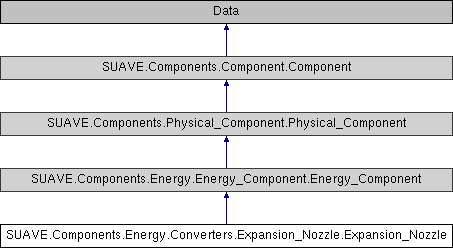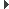SUAVE  2.5.2 An Aerospace Vehicle Environment for Designing Future AircraftSUAVE.Components.Energy.Converters.Expansion_Nozzle.Expansion_Nozzle Class Reference
Inheritance diagram for SUAVE.Components.Energy.Converters.Expansion_Nozzle.Expansion_Nozzle:## Public Member Functions

def __defaults__ (self)

def compute (self, conditions)

## Public Attributes

tag

polytropic_efficiency

pressure_ratioPublic Attributes inherited from SUAVE.Components.Energy.Energy_Component.Energy_Component
inputs

outputsPublic Attributes inherited from SUAVE.Components.Physical_Component.Physical_Component
tag

mass_properties

origin

symmetricPublic Attributes inherited from SUAVE.Components.Component.Component
tag

origin

generative_design_max_per_vehicle

generative_design_characteristics

generative_design_special_parent

## Static Public Attributes

gamma = conditions.freestream.isentropic_expansion_factor

Cp = conditions.freestream.specific_heat_at_constant_pressure

Po = conditions.freestream.pressure

Pto = conditions.freestream.stagnation_pressure

Tto = conditions.freestream.stagnation_temperature

R = conditions.freestream.gas_specific_constant

Mo = conditions.freestream.mach_number

Tt_in = self.inputs.stagnation_temperature

Pt_in = self.inputs.stagnation_pressure

pid = self.pressure_ratio

etapold = self.polytropic_efficiency

Pt_out = Pt_in*pid

Tt_out = Tt_in*pid**((gamma-1)/(gamma)*etapold)

ht_out = Cp*Tt_out

Mach = np.sqrt((((Pt_out/Po)**((gamma-1)/gamma))-1)*2/(gamma-1))

float i_low = Mach < 1.0

float i_high = 1.0

float P_out = 1.0 *Mach/Mach

T_out = Tt_out/(1+(gamma-1)/2*Mach*Mach)

h_out = Cp*T_out

u_out = np.sqrt(2*(ht_out-h_out))

float rho_out = P_out/(R*T_out)

tuple area_ratio = (fm_id(Mo,gamma)/fm_id(Mach,gamma)*(1/(Pt_out/Pto))*(np.sqrt(Tt_out/Tto)))

stagnation_temperature

stagnation_pressure

stagnation_enthalpy

mach_number

static_temperature

density

static_enthalpy

velocity

static_pressure

area_ratio

## Detailed Description

```This is a nozzle component intended for use in expansion.
Calling this class calls the compute function.

Assumptions:
Pressure ratio and efficiency do not change with varying conditions.
Subsonic or choked output.

Source:
https://web.stanford.edu/~cantwell/AA283_Course_Material/AA283_Course_Notes/
```

## ◆ __defaults__()

 def SUAVE.Components.Energy.Converters.Expansion_Nozzle.Expansion_Nozzle.__defaults__ ( self )
```This sets the default values for the component to function.

Assumptions:
None

Source:
N/A

Inputs:
None

Outputs:
None

Properties Used:
None
```

Reimplemented from SUAVE.Components.Energy.Energy_Component.Energy_Component.

## ◆ compute()

 def SUAVE.Components.Energy.Converters.Expansion_Nozzle.Expansion_Nozzle.compute ( self, conditions )
```This computes the output values from the input values according to
equations from the source.

Assumptions:
Constant polytropic efficiency and pressure ratio
If pressures make the Mach number go negative, these values are corrected

Source:
https://web.stanford.edu/~cantwell/AA283_Course_Material/AA283_Course_Notes/

Inputs:
conditions.freestream.
isentropic_expansion_factor         [-]
specific_heat_at_constant_pressure  [J/(kg K)]
pressure                            [Pa]
stagnation_pressure                 [Pa]
stagnation_temperature              [K]
specific_gas_constant               [J/(kg K)]
mach_number                         [-]
self.inputs.
stagnation_temperature              [K]
stagnation_pressure                 [Pa]

Outputs:
self.outputs.
stagnation_temperature              [K]
stagnation_pressure                 [Pa]
stagnation_enthalpy                 [J/kg]
mach_number                         [-]
static_temperature                  [K]
static_enthalpy                     [J/kg]
velocity                            [m/s]
static_pressure                     [Pa]
area_ratio                          [-]
denisty                             [kg/m^3]

Properties Used:
self.
pressure_ratio                      [-]
polytropic_efficiency               [-]
```

The documentation for this class was generated from the following file:
• /Users/emiliobotero/Dropbox/SUAVE/SUAVE/trunk/SUAVE/Components/Energy/Converters/Expansion_Nozzle.py